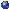Fixed point Floer Homology was introduced by A. Floer in his proof of the Arnold conjecture for monotone symplectic manifolds. In the first half of the talk we will briefly introduced the machinery of Floer Homology, while in the second half we will compute the Floer Homology of a Dehn twist on a surface following the work of P. Seidel. Time permitting at the end we will state a generalisation of such computations for monotone symplectic manifolds based on some ideas borrowed from Symplectic Field Theory. This is a candidacy talk